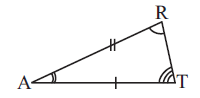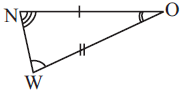# Ex.7.2 Q5 Congruence of Triangles - NCERT Maths Class 7

Go back to  'Ex.7.2'

## Question

In the figure, the two triangles are congruent. The corresponding parts are marked. We can write $$ΔRAT ≅$$?Video Solution
Congruence Of Triangles
Ex 7.2 | Question 5

## Text Solution

What is known?

The given two triangles are congruent and their corresponding parts are marked on the given figure.

$$\overline{NO}\text{ }=\text{ }\overline{AT}$$ , $$\overline{AR}\text{ }=\text{ }\overline{OW}$$

$$∠W = ∠R, ∠A = ∠O$$ and $$∠T = ∠N$$

What is the unknown?

Congruence of $$ΔRAT$$?

Reasoning:

This question is based on the concept of congruence of two triangle when two angles and one side (or two angles and one side) of the triangles are equal. If two angles and one side of a triangle are equal to the corresponding two angles and one side of another triangle then the two triangles can be congruent by $$ASA$$ Congruence criterion, by using this criterion you can find out the triangle congruent to $$RAT$$.

Steps:

From the figure, it can be observed that

\begin{align} \angle RAT &=\angle WON \\\angle ART & =\angle NOW \\ \overline{AR}\text{ } &=\text{ }\overline{OW}\end{align}

Therefore, $$ΔRAT ≅$$ WON (By $$ASA$$ congruence criterion)

Learn from the best math teachers and top your exams

• Live one on one classroom and doubt clearing
• Practice worksheets in and after class for conceptual clarity
• Personalized curriculum to keep up with school